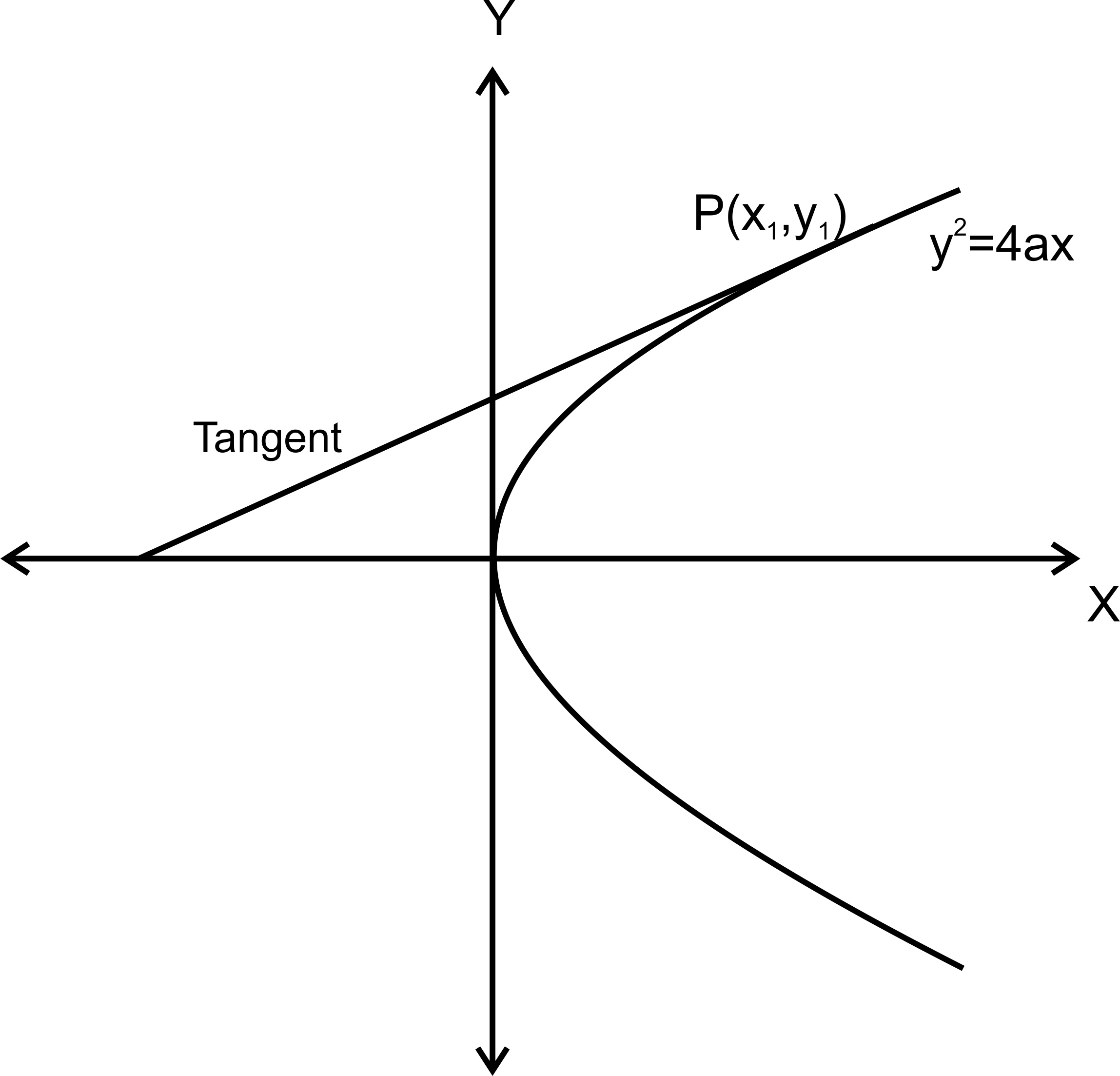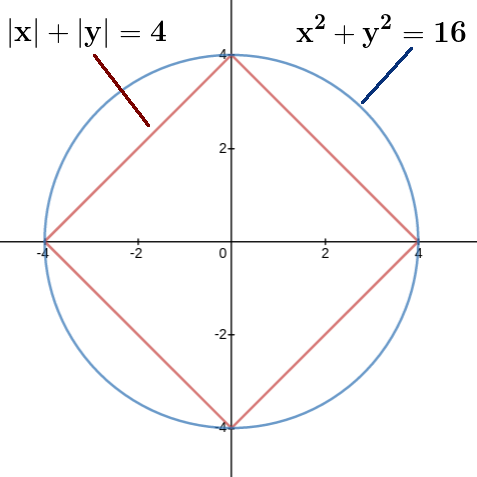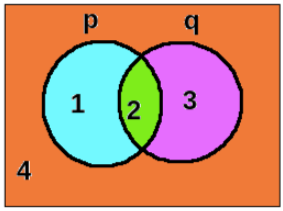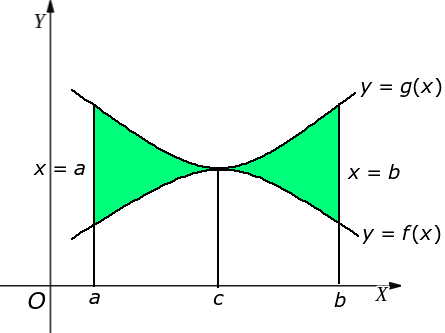Filter By

7/12

225

## Crack CUET with india's "Best Teachers"

• HD Video Lectures
• Unlimited Mock Tests
• Faculty Support#### If one end of a focal chord AB of the parabolais atthen the equation of the tangent to it at B is : Option: 1Option: 2Option: 3Option: 4Length of the Latus rectum and parametric form -

Parametric Equation:

From the equation of the parabola, we can write-

Tangents of Parabola in Point Form -

Tangents of Parabola in  Point Form-Correct Option 3

Option: 3

## Crack NEET with "AI Coach"

• HD Video Lectures
• Unlimited Mock Tests
• Faculty Support#### If  for  then Option: 1Option: 2Option: 3Option: 4Use

Correct Option (3)

#### Ifbe a complex number satisfyingthencannot be :  Option: 1Option: 2Option: 3Option: 4Complex number -

A complex number is the sum of a real number and an imaginary number. A complex number is expressed in standard form as a + bi where a is the real part and b is the imaginary part. For example, 5 + 2i is a complex number. So, too, is 3 + 4i√3.We write the complex number by C or z = a + ib, a and b are real number (a, b ∈ R).

• a is real part of the complex number and denoted by Re(z),

• b is the imaginary part of the complex number and denoted by Im(z),

E.g :    z = 2 + 3i is a complex number.

With Re(z) = 2 and Im(z) = 3

-

Area of triangle, circle (formula) -

Equation of Circle:

The equation of the circle whose center is at the pointand have radius r is given byIf the center is origin then,, hence equation reduces to |z| = r

Interior of the circle is represented byThe exterior is represented byHere z can be represented as x + iy andis represented by-

z = x + iy

|x| + |y| = 4Minimum value of |z| =Maximum value of |z| = 4So |z| can't beCorrect Option (1)

## Crack JEE Main with "AI Coach"

• HD Video Lectures
• Unlimited Mock Tests
• Faculty Support#### The length of the minor axis (along y-axis) of an ellipse in the standard form isIf this ellipse touches the line,then its eccentricity is :  Option: 1Option: 2Option: 3Option: 4What is Ellipse? -

Ellipse

Standard Equation of Ellipse:

The standard form of the equation of an ellipse with center (0, 0) and major axis on the x-axis is1. a > b

2.  the length of the major axis is 2a

3.  the coordinates of the vertices are (±a, 0)

4.  the length of the minor axis is 2b

5.  the coordinates of the co-vertices are (0, ±b)

-

Equation of Tangent of Ellipse in Parametric Form and Slope Form -

Slope Form:-Correct Option (2)

#### Ifis false, then the truth values of p and q are respectively :   Option: 1 F, TOption: 2 T, F Option: 3 F, F Option: 4 T, T

Relation Between Set Notation and Truth Table -

Sets can be used to identify basic logical structures of statements. Statements have two fundamental roles either it is true or false.

Let us understand with an example of two sets p{1,2} and q{2,3}.Using this relation we get-

Practise Session - 2 -

Q1. Write the truth table for the following statement pattern:-Correct Option (4)

## Crack CUET with india's "Best Teachers"

• HD Video Lectures
• Unlimited Mock Tests
• Faculty Support#### The following system of linear equationshas  Option: 1 infinitely many solutions,satisfyingOption: 2 infinitely many solutions,satisfyingOption: 3 no solution Option: 4 only the trivial solution.

System of Homogeneous linear equations -If ? ≠ 0, then x= 0, y = 0, z = 0 is the only solution of the above system. This solution is also known as a trivial solution.

If ? = 0, at least one of x, y and z are non-zero. This solution is called a non-trivial solution.

Explanation: using equation (ii) and (iii), we haveThis is the condition for a system have Non-trivial solution.

-so infinite non-trivial solution exist

now equation (1) + 3 equation (3)

10x - 20z = 0

x = 2z

Correct Option 2

#### Given :andThen the area (in sq. units) of the region bounded b the curves,andbetween the lines,is :  Option: 1Option: 2Option: 3Option: 4Area Bounded by Curves When Intersects at More Than One Point -

Area bounded by the curves  y = f(x),  y = g(x)  and  intersect each other in the interval [a, b]

First find the point of intersection of these curves  y = f(x) and  y = g(x) , let the point of intersection be x = cWhen two curves intersects more than one point

rea bounded by the curves  y=f(x),  y=g(x)  and  intersect each other at three points at  x = a, x = b amd x = c.

To find the point of intersection, solve f(x) = g(x).

For x ∈ (a, c), f(x) > g(x) and for x ∈ (c, b), g(x) > f(x).

Area bounded by curves,